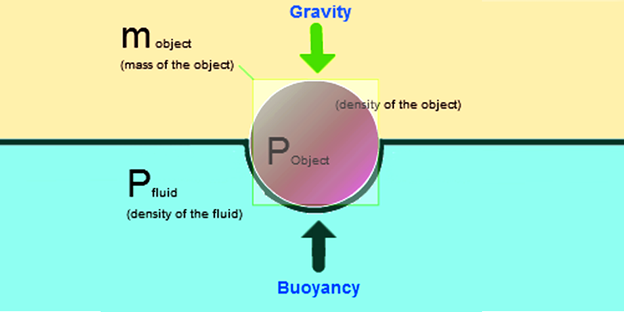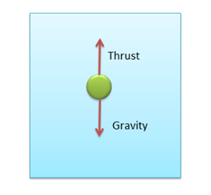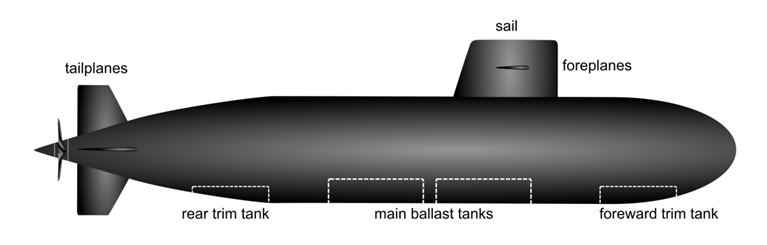# Buoyant Force

We often observe that our body feels lighter while taking a swim in the pool. Also, when drawing water from a well, the bucket feels lighter till it is partially or fully immersed in water. Have you ever wondered why this change in weight is felt?

We can say, when immersed in water or any other fluid, the body experiences a force from the downward direction opposite to the direction of the gravitational pull, which is responsible for the decrease in its weight. It can be the reason why a ball of plastic floats in water rather than sinking to the base by its weight. But here too, we observe that some objects such as a plastic bottle float over water, while others such as a needle sink. In this article, we shall learn about the concept of buoyancy and buoyant force which gives a proper explanation of such observations.

## What is Buoyancy?

Buoyancy is the force that causes objects to float. It is the force exerted on an object that is partly or wholly immersed in a fluid. Buoyancy is caused by the differences in pressure acting on opposite sides of an object immersed in a static fluid. It is also known as the buoyant force. Buoyancy is the phenomena due to Buoyant Force.

We can define Buoyancy as:

The upward force applied by the fluid on the object or the body when an object is put in or submerged in the fluid.

### Unit:

The unit of the buoyant force is the Newton (N).

When a body is in water, it displaces some amount of water owing to its weight. The amount of displaced water is computed by the object’s density which relates to volume. The volume of a cricket ball and beach ball can be the same as their different weight their density differs. The solid steel block descends in water but the steel ship does not. Therefore, the quantity of displaced water in the upward direction is elucidated in terms of Buoyant force. Let’s discuss the buoyancy force and its formulation with diverse devices grounded on it.

### What is Buoyant Force?

When an object is immersed in a liquid it experiences an upward force known as Buoyant Force. This phenomenon of experiencing an upward force is known as Buoyancy.

It is as an upward force exerted by a fluid that opposes the weight of an object immersed in a fluid. As we know, the pressure in a fluid column increases with depth. Thus, the pressure at the bottom of an object submerged in the fluid is greater than that at the top. The difference in this pressure results in a net upward force on the object which we define as buoyancy.### Force of Buoyancy:

When we submerge an object in a fluid, an upward force is experienced by the object. This force is applied by the fluid on the object which makes it to, rise up and is called the Force of Buoyancy. The scale of this force is precisely equal to the amount or weight of the liquid displaced.### Center of Buoyancy:

The point where the force of Buoyancy is applied or the point on the object where the force acts are termed as the Center of Buoyancy. It should be illustrated that the force of buoyancy is a vertical force, and thus, the Center of Buoyancy is the point situated on the centre of the gravity of the liquid that is being displaced by the object submerged.

You may also want to check out these topics given below!

## Why does an object float or sink in water?

If one considers a single column of liquid, then it can be considered as a concoction of the many overlying layers, one over the other with fluctuating pressure. The pressure at the bottom of the liquid would be greater than at the top; this is for the reason that as we go down in the liquid the number of layers of the liquid that are overlying i.e. the layers one over the other, increases.

Owing to the difference in the pressure amid the layers, there tends to be a made-up force being applied to it in the upward direction. This force leads to the acceleration of the object that has been submerged, in the upward direction. The force is always in the vertical direction.

It can also be said that the magnitude of the upward force is basically equivalent to the difference in the pressure of the topmost and the last layer and also equivalent to the weight of the fluid that has been displaced.

Floating is the consequence of the above concept. The object should be less dense than water, or else, if its density is greater, it will have a tendency to sink.

### Density and Relative Density

In order to understand the concept of buoyancy properly, let us understand the concept of density and relative density.

#### Density:

The density of a material is defined as its mass per unit volume. It’s a measurement of how tightly matter is packed together.

In numerical terms, density is defined as:

$Densit, \rho =\frac{Mass}{Volume}=\frac{M}{V}$

• The SI unit of Density is measured using kilogram per cubic metre (kg/m3).
• The density is 0.9584 grams per cubic centimetre at 100° Celsius.

#### Relative Density:

The relative density of a substance is defined as the ratio of the density of the substance to the density of water at C. Relative density is also known as the specific gravity of the substance.

$Relative\; Density = \frac{Density \: of \: a \: substance}{Density\: of \: water \: at\: 4^{\circ}C}$

Since relative density is a ratio of similar quantities, it has no unit.

## What is Upthrust?

As discussed, the buoyant force is the upward force exerted on an object that is wholly or partly immersed in a fluid. This upward force is also called as Upthrust. It is due to the buoyant force that a body submerged partially or wholly in a fluid appears to lose its weight i.e. appears to be lighter.An object whose density is greater than that of the fluid in which it is submerged tends to sink. If the object is either less dense than the liquid or is shaped appropriately (as in a boat), the force can keep the object afloat. In terms of relative density, if the relative density of a substance is less than one it floats in water and substances with a relative density greater than 1 sink in water.

The buoyant force depends on:

• The volume of the body immersed which is equal to the volume of fluid displaced.
• The density of the fluid.

## Applications of Buoyancy

It is due to buoyancy that human swimmers, fish, ships, and icebergs stay afloat. Some applications of buoyancy are given in the points below.

### Submarine:

A submarine has a large ballast tank, which is used to control its position and depth from the surface of the sea. A submarine submerges by letting water into the ballast tank so that its weight becomes greater than the buoyant force.Submarine

### Hot Air Balloon:

The atmosphere is filled with air that exerts buoyant force on any object. A hot air balloon rises and floats due to the buoyant force. It descends when the balloon’s weight is higher than the buoyant force. It becomes stationary when the weight equals the buoyant force.Hot Air Balloon

### Ship:

A ship floats on the surface of the sea because the volume of water displaced by the ship is enough to have a weight equal to the weight of the ship. A ship is constructed in a way so that the shape is hollow, to make the overall density of the ship lesser than the sea water. Therefore, the buoyant force acting on the ship is large enough to support its weight.

### Fish:

A certain group of fishes uses Archimedes’ principle to go up and down the water. To go up to the surface, the fishes will fill its swim bladder (air sacs) with gases (clever isn’t it?). The gases diffuse from their own body to the bladder and thus making the body lighter. This enables the fishes to go up

## Frequently Asked Questions on Buoyant Force

### Why is buoyancy important in swimming?

Buoyancy is important in swimming because it helps the swimmer to stay closer to the surface. This is because the pressure experienced by the swimmer under the water is more than the pressure experienced above. This is also the reason why swimmers can float on the surface of the water.

### What causes buoyancy?

The pressure exerted by the fluid in which the object is immersed causes the buoyancy. Also, the buoyant force experienced by the object is always upwards because the pressure of the fluid increases with the depth.

### What are the three types of buoyancy?

The three types of buoyancy are positive buoyancy, negative buoyancy, and neutral buoyancy.

Positive buoyancy is when the immersed object is lighter than the fluid displaced and this is the reason why the object floats.

Negative buoyancy is when the immersed object is denser than the fluid displaced which results in the sinking of the object.

Neutral buoyancy takes place when the weight of immersed object is equal to the fluid displaced. Dive taken by the scuba diver is an ideal example for neutral buoyancy.

### What are the examples of buoyancy?

The following are some of the examples of buoyancy:

• A boat or a ship floating in water is an example of buoyancy.
• Immersing a cork in water will result in floating of it on the surface and this is because of the buoyancy.

### How are buoyancy and density related?

Buoyancy or the buoyant force is directly proportional to the density of the immersed fluid.